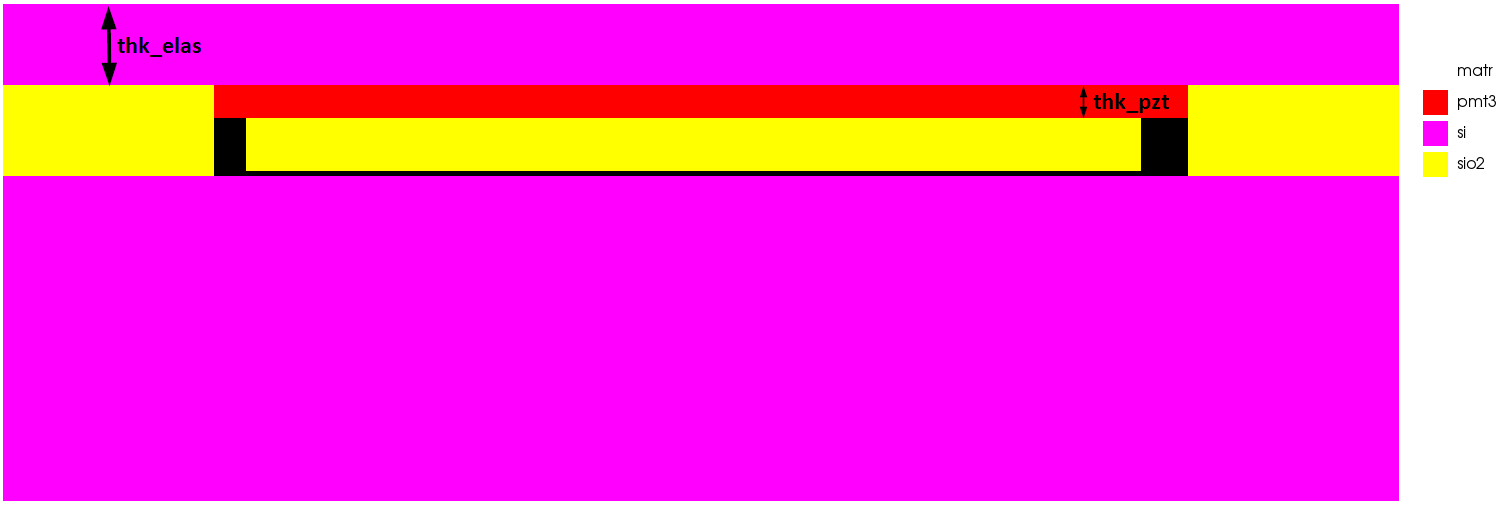# PMUT Unit Cell Monte Carlo Study

Monte Carlo Simulation a statistical method which uses simulation to model the probability of outcomes of a complex model whose behaviour cannot be easily determined due to a vast number of variables. PMUT performance is affected by a number of design variables.

In this example, we vary both the Silicon thickness and the PZT thickness of a PMUT cell to get a better idea of the design space and how the key performance (KPIs) relate to these inputs.

## Model Setup

A schematic of the model and the two input variables can be seen below:The key model parameters were as follows. Silicon thickness and PZT thickness are set as design variables to be randomly varied (indicated by *)

Design Variable Description Default Value
width_pitch_x Pitch 43 um
thk_sub Substrate thickness 10 um
thk_cav Cavity thickness 1.8 um
thk_pzt* PZT thickness 1 um
thk_elas* Silicon thickness 2.5 um

2000 random input variables were created with the following constraints:

• Silicon thickness: 2.5 um ± 2.5%
• PZT thickness: 1 um ± 5%

## Monte Carlo Results

The full study was completed in 9 minutes when using 2 cores per simulation and had a total cost of 14.39 Core-Hours.

Using the outputs from the simulations, it is possible to calculate the KPIs such as centre frequency, membrane displacement and frequency bandwidth. The inputs and outputs can then be plot in a number of ways to get insight into the device performance. Results are plotted below in MATLAB.

## Try this Yourself

To run this Monte Carlo study you will need to download the OnScale and MATLAB files.